Next: Conclusions Up: Image Restoration and Super-Resolution Previous: Introduction

# Image and Spectrum Recovery

## General Procedure

The procedure used to recover both spectra and images or, alternatively, to map from one type of PSF to another, is the same and is outlined below:

1. Generate a model instrumental point spread function or obtain an observed one.
2. Determine the net instrument response desired. That is, determine what
, the effective PSF of the extended instrument should be.
3. Use a numerical algorithm, the convolution network (discussed in detail below), to search for the solution that provides the desired mapping of the observed data to the desired form. That is, find asuch thatis the desired extended instrument PSF. Note that the extended instrument is implemented totally independent of the specific output data of the instrument, i.e.,is data independent.
4. Apply the convolution network's solution to the observations to be mapped, i.e., compute
.

## HST-Like and HST Image Reconstruction

We first made preliminary studies investigating recovery of images that have been broadened by the HST's mirror by modeling its PSF as shown in Fig. 1. At left is a simple PSF based on a superposition of two Gaussians, and at right is a typical Tiny TIM PSF for HST's Wide Field/Planetary Camera.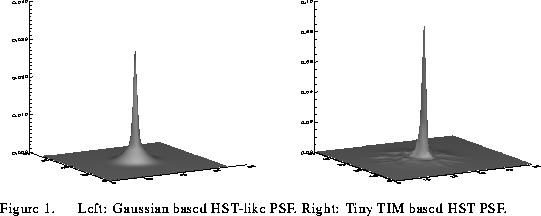The results of applying our procedure outlined above are given in Fig. 2. At left is the original ground-based image of M82. The middle image shows the M82 data degraded by our model PSF. At right is the recovered estimate of the image using the procedures outlined above. Note that the estimated image does not use any knowledge of the original image. Fig. 3 shows how well the recovery process works in the presence of artificially added noise.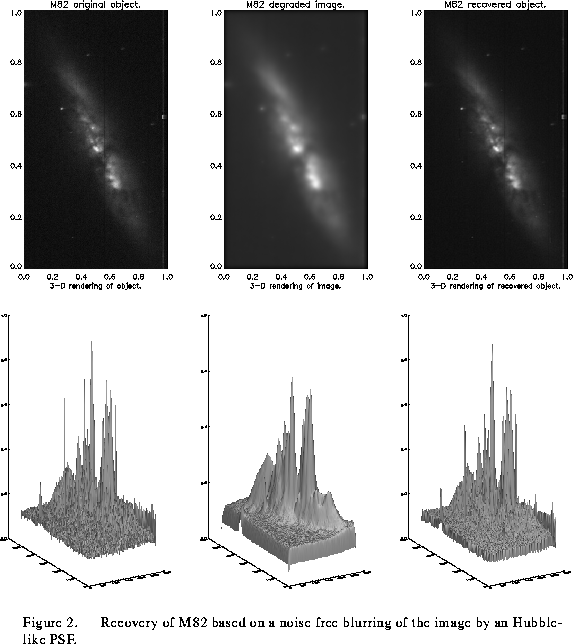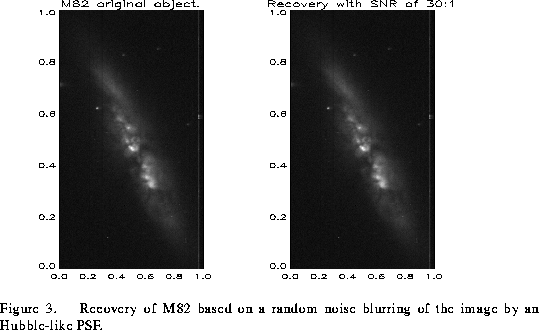We have also applied our recovery procedure to HST images accessible from the ST ScI image restoration test data sets. Our results for HST images are shown in the following figures. Fig. 4 is an HST image of Jupiter, our enhancement, and a comparison with a Richardson-Lucy enhancement (provided by Bob Hanisch). The PSF used is a Tiny Tim model PSF. Fig. 5 shows our recovery of an HST image of Saturn using an observed 53x53 point HST PSF. Fig. 6 is based on an IRAF M51 image from the ST ScI test data set. The Tiny Tim-PSF processed image used as a starting point in our processing is also from the image restoration test data set. Our recoveries from test images with and without noise are shown with a Richardson-Lucy recovery with noise (also from the ST ScI test data set). We believe that our method compares well with the Richardson-Lucy method, but have not made any quantitative comparisons.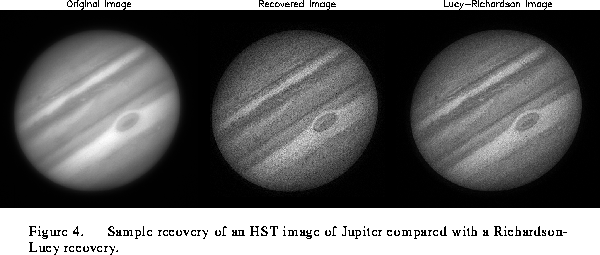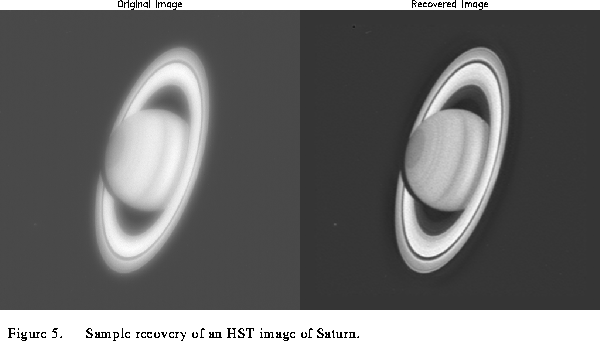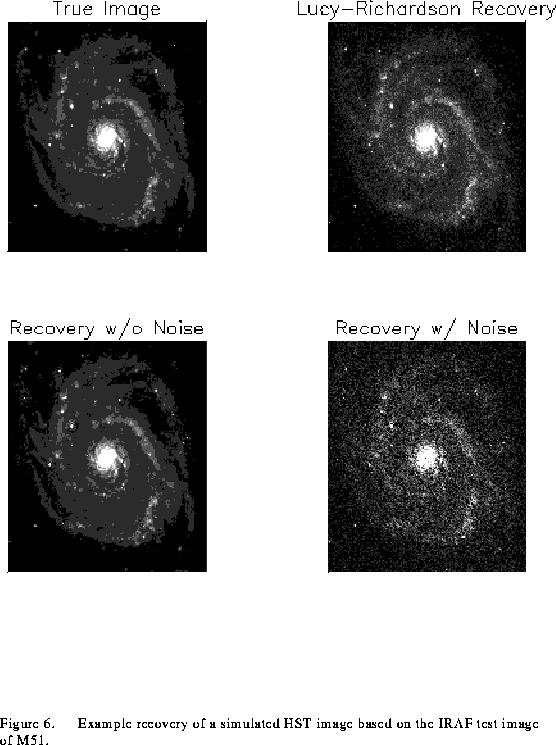## Recovery of Spectra

### Tunable Diode Laser Spectroscopy

Our recovery procedure was applied also to spectral data, in this case to a tunable diode laser (TDL) spectrum. The TDL observation of 13
m propane is shown in Fig. 7. The second row shows an example of a constrained signal space deconvolution of this spectrum, using a nonlinear method (after Blass and Halsey 1981 and Jansson 1984). The other plots are examples of resolution enhancement using the our procedure with the target function being Gaussians having a full width at half maximum (FWHM) of 2 data points, 3 data points, and a unit impulse function, respectively. The TDL PSF is modeled with a Gaussian having a FWHM of 6 data points. The use of targeted PSFs is discussed in more detail below.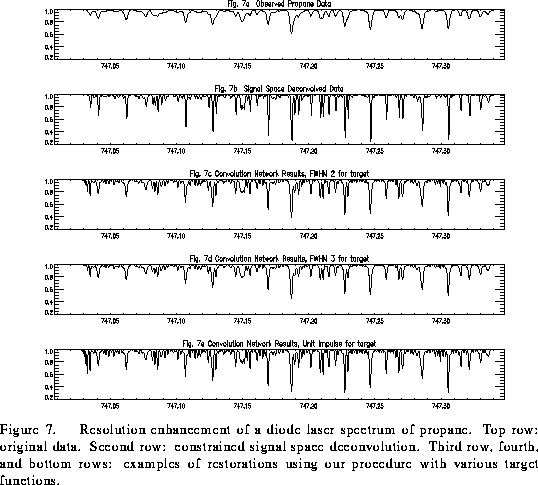### Numerical Apodization

Fig. 8 shows that it is practical to map a spectrum from a sinc apodization to a positive semi-definite distribution using our numerical procedure. A sinc point spread function (top, Fig. 8) is mapped to a sincfunction (middle, Fig. 8), and to a Gaussian function (bottom, Fig. 8) using the linear filters derived from our procedure.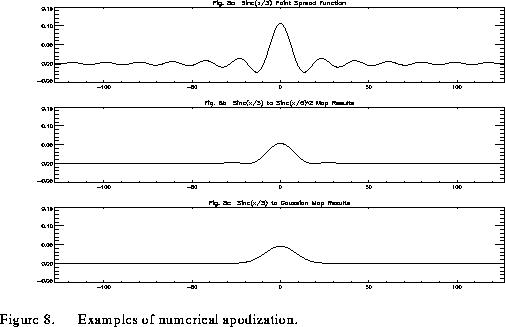### Numerical Apodization Plus Spectral Recovery: FTS Spectrum

One benefit of applying our extended instrument model is the ability to cascade a series of filter functions to achieve a desired end result. For example, we can model the response of a Fourier Transform Spectrometer (FTS) spectrum whose PSF is a sinc function and to map its response to a sincapodized spectrum using our procedure. The numerically apodized spectrum was then deconvolved (restored) using a nonlinear constrained signal space deconvolution algorithm. The ``true'' spectrum (before observation) is shown at the top of Fig. 9. The second row of Fig. 9 shows an observation from a simulated FTS spectrometer. The third row shows the sincapodized spectrum. An estimate of the true spectrum derived from the apodized spectrum is shown in the bottom row by applying a further inverse filter.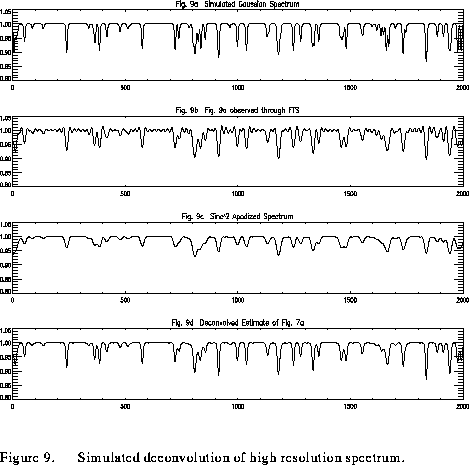## Deconvolution by a Novel Neural Network

The instrumental convolution is characterized by Eq. 1, a Fredholm integral equation of the first kind. In principle, one can analytically solve forby using Fourier transform theory. The instrument model equation is
and its Fourier Transform then is
. Rearranging and using the inverse Fourier Transform, one recovers an estimate of
This direct inversion has serious problems:
is not well behaved, in particular it amplifies noise. The convolution network searches for an approximatethat minimizes an error function,
, where
is the target function. The convolution network is derived from the error-backpropagation neural network model with a slightly more complex, yet more natural connection scheme for imaging systems. Many deconvolution algorithms use a correction term, e, of the form:
The convolution network involves a correction that has the form:
where
is the point spread function and
is the desired result of the mapping (i.e., for a sinc
function mapped to a sinc
function,is the sincfunction,is a sincfunction, andis the function that minimizes). After determining, the application of the convolution network is computationally inexpensive since it involves only a convolution. It is feasible to build an instrument that can be tailored for real-time data enhancement/processing via several filter functions,
, as shown in Fig. 6.
Next: Conclusions Up: Image Restoration and Super-Resolution Previous: Introduction

rlw@sundog.stsci.edu
Mon Apr 18 12:13:37 EDT 1994